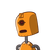# Q6. Two points A and B are (0, 7) and (6, 9) respectively. (a) Find the gradient of the line AB.(b) The eq

Q6. Two points A and B are (0, 7) and (6, 9) respectively.

(a) Find the gradient of the line AB.

(b) The equation if the line AB is x + Py + Q = 0. Find P and Q.

(c) If C (12,2) is the midpoint of BM, find the coordinates of M

(d) Calculate AC

(e) A fourth point D lies on the line AB. The line CD is parallel Find the coordinates of D​

### 1 thought on “Q6. Two points A and B are (0, 7) and (6, 9) respectively. <br /><br />(a) Find the gradient of the line AB.<br /><br />(b) The eq”

1.